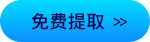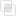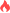python中subplot函数怎么画图？

Python2021-04-19 15:56:24816浏览 · 0收藏 · 0评论1、说明

（1）调用subplot()函数可以创建子图，程序可以在子图上绘制。subplot(nrows、ncols、index、**kwargs)函数的nrows参数指定将数据图区域分成多少行，ncols参数指定将数据图区域分成多少列，index参数指定获得多少区域。

（2）subplot()函数还支持直接输入一个三位数的参数，其中第一位数是nrows参数；第二位数是ncols参数；第三位数是index参数。

2、参数

nrows:  subplot的行数

ncols： subplot的列数

sharex ：所有subplot应该使用相同的X轴刻度(调节xlim将会影响所有的subplot)

sharey: 所有subplot应该使用相同的Y轴刻度(调节ylim将会影响所有的subplot)

subplot_kw: 用于创建各subplot的关键字字典

**fig_kw: 创建figure时的其他关键字

3、实例

```import numpy as np
import matplotlib.pyplot as plt
x = np.arange(0, 100)
#作图1
plt.subplot(2,2,1)  #等效于plt.subplot(221)
plt.plot(x, x)
#作图2
plt.subplot(2,2,2)
plt.plot(x, -x)
#作图3
plt.subplot(2,2,3)
plt.plot(x, x ** 2)
plt.grid(color='r', linestyle='--', linewidth=1,alpha=0.3)
#作图4
#plt.subplot(224)
#plt.plot(x, np.log(x))
plt.show()```python WSGI规范是什么python整数的进制转换python中Queue如何通信python中进程池Pool的初始化python Pool常用函数有哪些Newton插值法——Python实现python机器学习常用包编程知识 | python基础语法从零开始学python---基础语法Python 列表List 的使用Python import 的用法python正则表达式re库详解python中figure()函数画两张图java反射调用指定的属性257

java获取class实例的方式635

java中反射提供哪些功能867